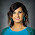## 3.07.2011

### Slopes of Parallel and Perpendicular Lines

Previously I had created what I thought was a pretty good investigation of slopes of parallel and perpendicular lines. It reviewed graphing slope-intercept form and point-slope form, plotting points, finding intersections, and comparing/contrasting equations with a nice ending that would smoothly transition into systems of equations. Little did I know, I made an error on one set of equations that made them neither parallel nor perpendicular. Another set of equations ran off of the graph, which made it harder to see that the lines were perpendicular rather than just intersecting. And I gave this to students on a day I was not in class. And weird timing things happened and we skipped a few days before going back to it. Probably not my best idea ever.

So alas, I scrapped it and found this packet on-line. It just seemed like my students were confused and needed the basics without the investigation. We spent two class periods on it and I gave some for homework. It was straightforward and hit the four concepts I wanted to leave them with: using point-slope formula to write an equation in slope-intercept form, use the slope formula and determine if the slopes are parallel/perpendicular/neither, solve two equations for y and compare their slopes, and write the equation of a line parallel/perpendicular to a given equation. After this packet, I did an index card review.

Here is card 1a and 1b.

Same concept, different numbers. Students worked in partners so that they had someone to ask if they struggled. After 4 minutes, the cards rotate. Now the students receive cards 2a and 2b.

But, if they flip it over, the answer to 1a and 1b is on the back. The idea is that students are able to self-check and not spend the whole hour working problems incorrectly. I saw this idea on Amy's blog and also at a ICTM conference.  Create stations and each station has the solution to the previous station. Amy mentioned that her students wanted to rotate cards instead of rotating desks. I thought this would be easier as well and had my students sit in a circle. I made 8 sets of cards (total of 16) and we only had 4 sets of partners (8 students total) so then they were working on sets 1a/1b, 2a/2b, 3a/3b, and 4a/4b. The problem came when we rotated cards. The students with 4a/4b now had 1a/1b when they really needed 5a/5b so that they could flip them over and see the answers. Things got messy quickly and I was juggling index cards all over the place. It took me two classes in a row to figure out how to remedy this. My last class has 16 students so my set of 8 would have worked but they are terrible at transition and we waste too much time and energy of mine trying to complete simple tasks.

Light bulb! I put the two cards under the document camera. The slower students worked to complete one card while faster students could complete two in the same amount of time. (Hello differentiation!) After the timer went off (4 minutes again), I showed the solutions to both cards and then on to the new set of problems. The class was very well-behaved and quiet- most worked diligently.

I liked doing this but I want to make the station idea work too. I think that next time I should make students rotate stations or I should make two sets of 4 cards. My smaller class could do one set and then the other if there was enough time. Then my class of 16 could combine the two sets and use them all. But that won't always work, depending on the class size.

I think that I accomplished what I set out to, even if the method was different than what I imagined. But what fun is building a boat if you aren't carrying your tools while swimming?

1.2.